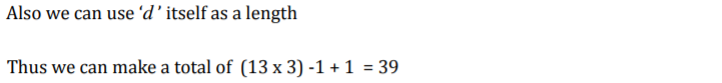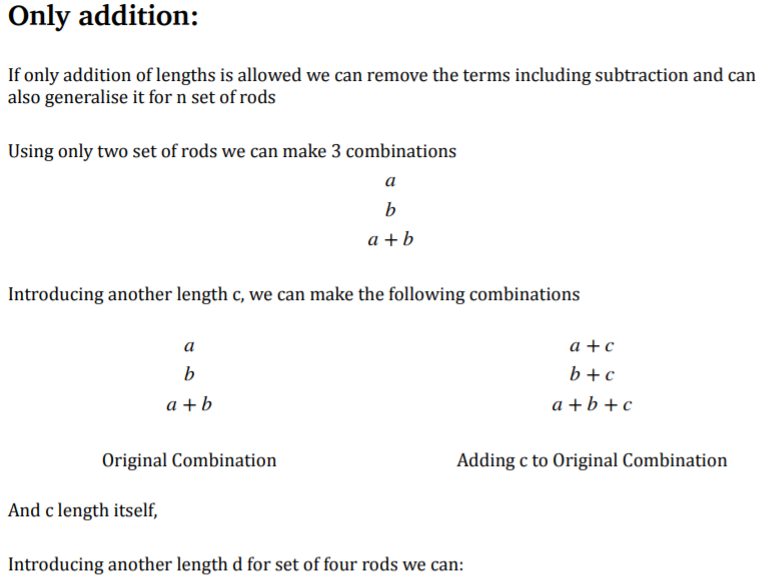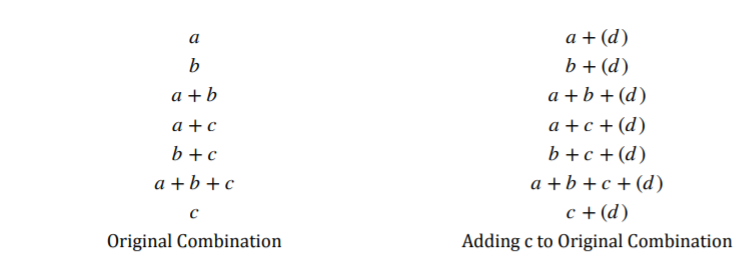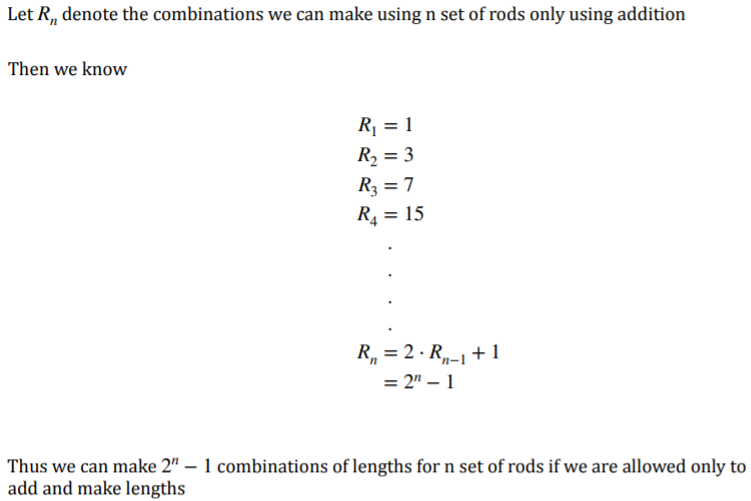#### You may also like### Pair Sums

Five numbers added together in pairs produce: 0, 2, 4, 4, 6, 8, 9, 11, 13, 15 What are the five numbers?### Summing Consecutive Numbers

15 = 7 + 8 and 10 = 1 + 2 + 3 + 4. Can you say which numbers can be expressed as the sum of two or more consecutive integers?### Big Powers

Three people chose this as a favourite problem. It is the sort of problem that needs thinking time - but once the connection is made it gives access to many similar ideas.

# Combining Lengths

##### Age 11 to 16Challenge Level

Elise from Hardwick Middle School in England found a set of 3 rods which can make the lengths from 1 to 7:
My solution to the counting rods problem was to use a set of rods with the lengths of 1, 2 and 4. By using these you can create the lengths 1 to 7. I found this out by using trial and error. I first tried it was 3, 4 and 5 just to give me an idea of the kind of numbers I would need. With these I couldn't create 1,2 or 6. To get 1 I figured I had to have a rod worth 1. I would also need one for 2.  Then it was for the third number. I went through the numbers and I could use 1 and 2 to get three but then I couldn't get any higher. This meant I had to use a four. If I had used three I could only make it to six but by using four I could get to seven.

Holly, Jack, Jake and Grace, Lilly from The Priory School, Shrewsbury in the UK all found the same way to get all of the lengths up to 9, which doesn't use 1 or 2. This is Holly's work: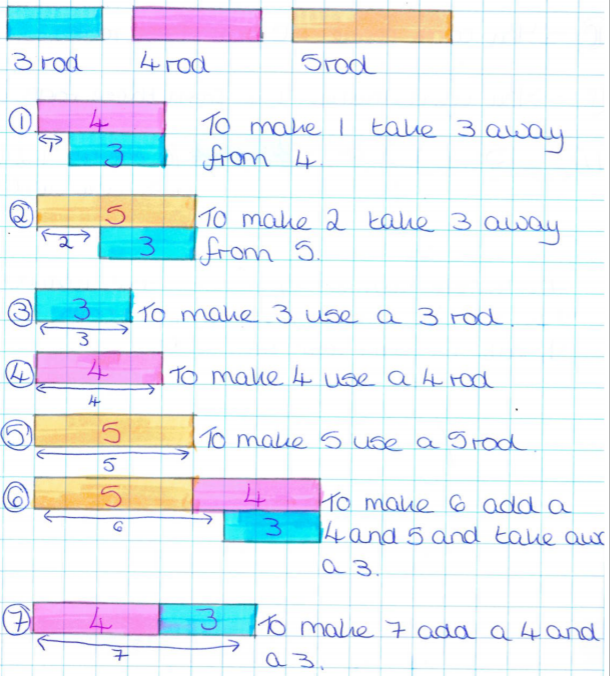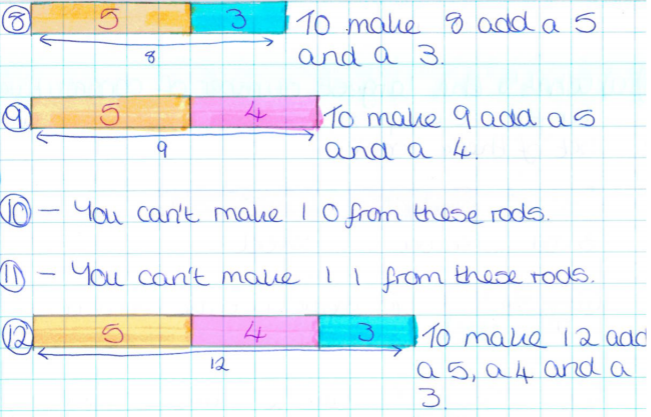Jack also used the lengths 1, 3 and 5 to make the lengths from 1 to 9 (click here to see Jack's work) and Grace and Jake used the lengths 2, 3, and 7 to make the lengths from 1 to 9 (click here to see Grace and Jake's work).

Douglass from Harrow International School Hong Kong described a systematic way to make sure that you have counted all of the different lengths you can make with your three chosen rods. In this way, Douglass convinced himself that you cannot make a length of 8 using rods of length 3, 4 and 6:
Turn the rods into numbers and of course we can make the length of the rod itself.
Then list them form the smallest to the largest, count the number it makes.
Take one of them out and count the number it makes, then replace while taking another one out. Repeat the process until all the rods have been taken out at least once. Then take two of them out each time and make sure they are a different pair each time, then three, then four till you finish the stage of taking out all the rods except one. Repeat the process again of instead of taking it away but it at the bottom and count the blank area.
This can be a very hard process but I have tried my best to think of an easier alternative.

Chris from Sha Tin College in Hong Kong found another set of 3 rods which can make the lengths from 1 to 9:
To create a value of 1-8 or 1-9, we must keep the same principle that there are two numbers that are 1 apart with a third number that has a higher value than the pair of numbers and to help fill in the missing numbers that the pair cannot create. An example of this would be 4,5 and 7 this combination of numbers would be capable of:
5 $-$ 4 = 1
7 $-$ 5 = 2
7 $-$ 4 = 3
4
5
7 + 4 $-$ 5 = 6
7
7 + 5 $-$ 4 = 8
5 + 4 = 9

Miss Warburton from Gems WSO (Wellington Academy Silicon Oasis) in UAE sent in the following list of sets of rods which can make all of the lengths from 1 to 10:
1,2,7
1,3,6
1,3,8
2,3,9
3,4,9
2,3,7

Justin from Sekolah Seri Cahaya in Malaysia found the following sets:
1, 5 and 7 can create numbers 1 to 11
2, 3 and 9 can create numbers 1 to 12

Tara and Chloe from Kings Dubai in UAE found:
9,1,3 go up to 13
1 = 1
2 = 3 $-$ 1
3 = 3
4 = 3 + 1
5 = 9 $-$ (1 + 3)
6 = 9 $-$ 3
7 = 9 + (1 $-$ 3)
8 = 9 $-$ 1
9 = 9
10 = 9 + 1
11 = (9 + 3) $-$ 1
12 = 9 + 3
13 = 9 + 3 + 1

Yiyi from Harrow International School in Hong Kong and Mahdi from Mahatma Gandhi International School in India both called the lengths of their rods $a, b$ and $c$ and then carefully chose values for them. This is Yiyi's work:
I have already chosen $1$ to be my first number as you can make ($b+1,c+1,b-1$ and $c-1$) already.
Then I have found more numbers we should and should not use. For example I thought that my second number shouldn't be $2$ because then when I add all of them together the number would be small, but I want to find a wide range numbers from it. $3$ can be also made if I include the number $4$ so now I have $1$ and $4.$ Now I can make $1, 4, 3 = 4-1,$ and $5 =4+1.$ However $6$ can't be made unless we have $7$ $(6=7-1), 10$ which is too big or $6$ itself.

If we use $6$ and do all the different ways above we get numbers $1,2,3,4,5,6,7,9,10,11$ - we are missing the number $8.$

However if we use $7$ as our third number we end up with a longer chain of numbers and they are all next to each other! We get $1,2,3,4,5,6,7,8$! (and $10,11,12$ which wouldn't count) We're just missing $9$!

Wobbuffet (Andrew) from GSAL in the UK also used logic to build a set of rods that can make all of the lengths from 1 to 10:
1, 2 or 3 will have to be used because if 4 was the smallest, it would have to be 4, 5, 7 or 4, 6, 7. The reason for
this is that there has to be differences of 1, 2 and 3. Each of them can't make [all of the lengths] from 1-10. 1 or 2 have to be used because otherwise, it has to go 3, 4, 6 or 3, 5, 6 (to make differences of 1 and 2). None of them make it from 1-10. If 2 and 3 are both used, there would be a difference of 1 and there is a third number
that could be any I like ($x$). Their total has to be at least 10. So the final number has to be at least 5.

5 isn't a good idea because 2+3=5 and it wouldn't get a free number for 2+3. 6 got from 1-9. It just wasn't able to make 10. 7 is bad as well because 7 $-$ 2=2+3. The $x-$2 could make something that couldn't be made with
another combination. If $x$=8, it isn't good either because 8 $-$ 3=2+3 (ends with the same reason as before). 9 does exceptionally well. This is one that I tried because I couldn't find any reason to hold it back. 9$\gt$2+3,
because 9 $-$ 2 $-$ 3 = 4. 4 can't be made in any other way. That is just good as it gets a combination that is rare with other sets. After testing, it got me from 1-12.

Jack tried using 4 rods and addition only: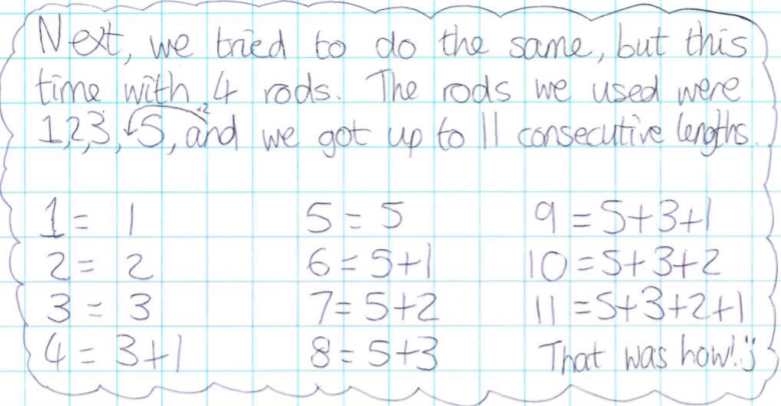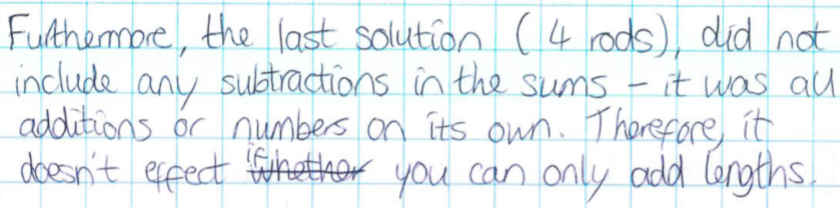Mia, Harry and Tom also tried adding only:
We started off by making different solutions where the rods had consecutive numbers as their lengths.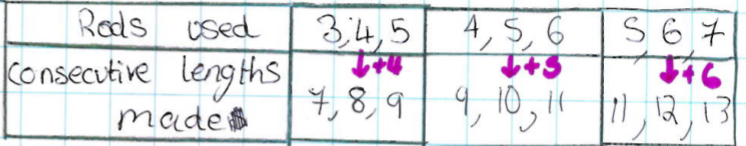So out prediction is that the rods 6, 7, 8 will be able to make the consecutive lengths 13, 14, 15, making the difference 7.

Back to the case with 3 rods, Billy from Otto-Hahn-Gymnasium Geesthacht in Germany and Ali from Deira International School in UAE used algebra to generate the best possible combination using 3 rods. This is some of Billy's work:
Let $a\lt b\lt c.$
In order to be able to combine the rods to the largest number of consecutive lengths, no combination should be "wasted", i.e. a certain combination should not be equal to another possible combination.

For $a,b,c$ there are the following combinations:
$a, b, c, a+b, a+c, b+c, c-b, c-a, b-a, c-a+b, c-b+a$ and either $c-b-a$ for $a+b\lt c$ or $a+b-c$ for $a+b\gt c.$

Billy chose the shortest rod first, and then the middle rod. Ali simply started with 2 rods and then built the problem up:
We begin by considering the first case of only two rods. What combination of lengths of two rods will allow us to measure the highest consecutive integer values?

For any values of two rods $(a,b),$ where $a\lt b,$ the possible combinations are:
$a,b,(b-a),(b+a)$
As we can see the maximum possible consecutive integers we can count up to with two rods is $4$ and the combination of $a$ and $b$ which allow that is $a=1$ and $b=3$:
$1,(3-1),3,(3+1)$
$=1,2,3,4$

Now we consider the case of three rods. To get the maximum possible measurable integer values we need to use the combination of the first two rods which give their maximum measuring ability which as we determined
above is $(1,3).$ So now we must find the third rod:

We have the three rods $(1,3,c).$ As we can see the maximum value the first two rods can give us is $4,$ which means $c$ must be the largest value obtainable that when $4$ is subtracted from, will give the next consecutive integer needed: $5.$
In other words:   $c-4=5$
This is because the largest possible value we can ever subtract from $c$ is $4$ and this has to be the next missing consecutive integer. Thus we determine $c=9.$
$(1,3,9)$ are the combinations of three rods which allow us to count to the maximum consecutive integers.

Nathan from Mossbourne Community Academy proved that making the lengths from 1 to 13 is the best you can do with 3 rods:
For subsequent numbers, we want to find the total amount of numbers that could potentially be made. Each number can be added, subtracted or not used at all. This gives $3$ options for every block, so there are $3^3=27$ possible numbers. How many of these use subtraction. Some negative numbers will be used, but these aren't helpful. If no blocks are used, $0$ is made. There are $27-1=26$ permutations using blocks.

Each combination could be reversed with the positive numbers negative and negative numbers positive to make $n$ into $-n.$ Let there be $a$ positive numbers made. There must also be $a$ negative numbers made. $2a=26$ so $a =13.$ The  maximum number possible is $13.$

Ali's method extends to $4$ or more rods very easily:
Repeating for $4$ rods, we have $(1,3,9,d).$ As before the $d$ must satisfy $d-13=14,$ because $13$ is the largest combination rods $1,3$ and $9$ can give us. Therefore $d=27$

We can see a pattern emerging:

$1$ rod = $(1)$
$2$ rods = $(1,3)$
$3$ rods = $(1,3,9)$
$4$ rods = $(1,3,9,27)$

It seems our optimum combination of rod lengths seem to follow a geometric progression of the form $3^{n-1}$ where $n$ is the number of rods we can pick.
To find the maximum integer we can count up to for each respective number of rods, we simply need to use the formula for the sum of a geometric progression... $S_n= \dfrac{3^{n - 1}}2$

Is this linked to Nathan's result?

Mahdi showed why the highest number that can be "counted" up to gets $3$ times bigger for each new rod, and found the corresponding pattern for addition only: